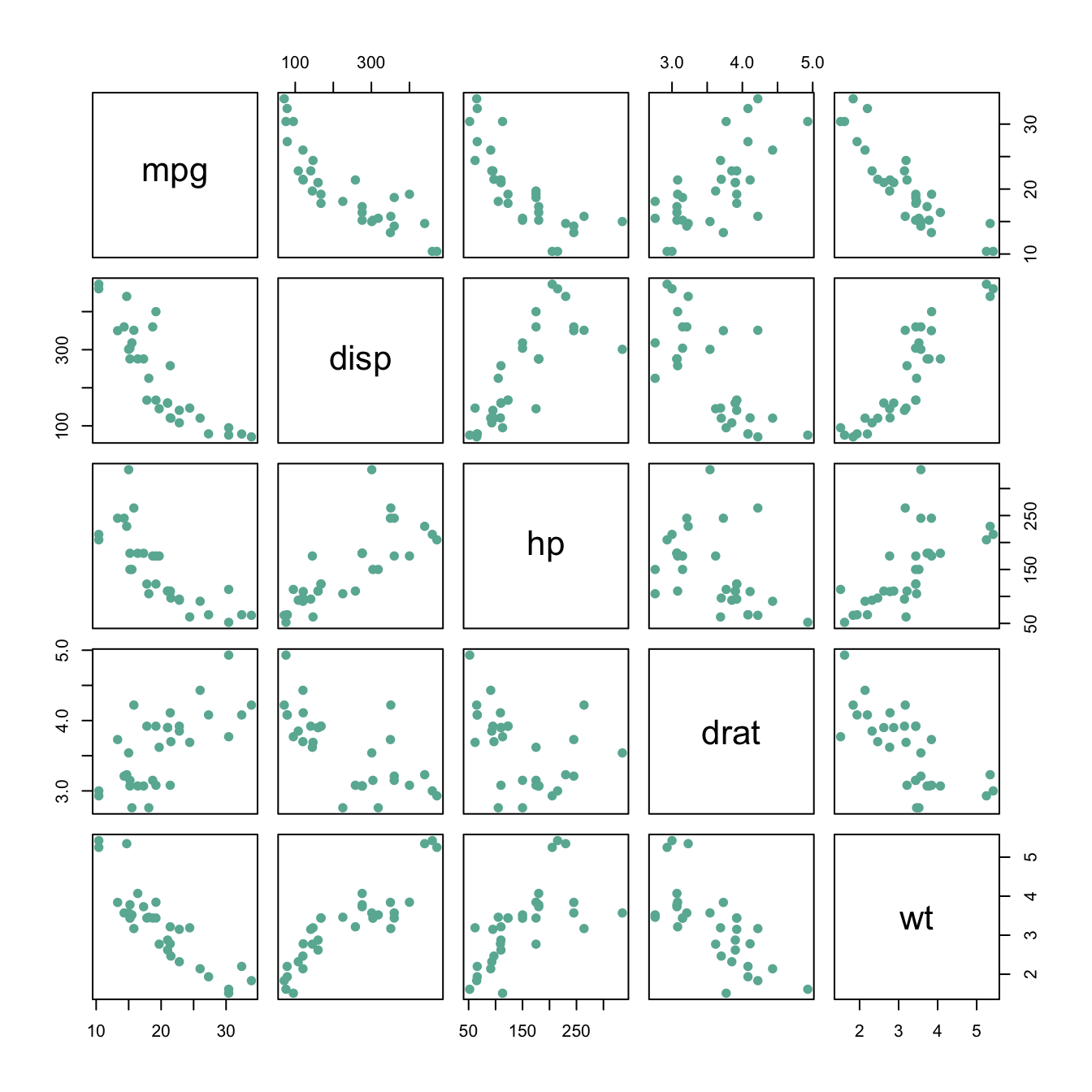# Basic scatterplot matrix

This post explains how to build a scatterplot matrix with base R, without any packages. It provides several reproducible examples with explanation and R code.

# Scatterplot matrix with the native `plot()` function

For a set of data variables (dimensions) X1, X2, ??? , Xk, the scatter plot matrix shows all the pairwise scatterplots of the variables on a single view with multiple scatterplots in a matrix format.

The native `plot()` function does the job pretty well as long as you just need to display scatterplots. For more option, check the correlogram section``````# Data: numeric variables of the native mtcars dataset
data <- mtcars[ , c(1,3:6)]

# Plot
plot(data , pch=20 , cex=1.5 , col="#69b3a2")``````

Related chart types

## Contact

This document is a work by Yan Holtz. Any feedback is highly encouraged. You can fill an issue on Github, drop me a message on Twitter, or send an email pasting yan.holtz.data with gmail.com.